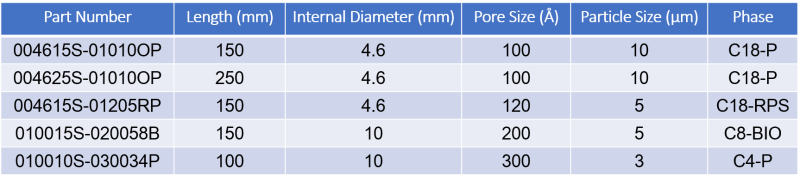Part Number

### DAISOGEL® columns can be ordered by desciption alone, i.e. "10mmIDx100mmL column of SP-120-5-ODS-BIO", or it can be ordered with a part number. The Part Number of a column is determined by the format shown below.

Format: AAAABBC-DDDEEFGG

AAAA: Column ID: 50mmID would be 0500; 4.6mmID would be 0046
BB: Column Length: 150mmL would be 15; 250mmL would be 25
C: (S) Screw-type, (F) Flange-type (See Analytical/ Preparative pages)
DDD: Silica Pore Size: 120A would be 012; 60A would be 006
EE: Particle Size: 10um would be 10; 5um would be 05
F: Particle Size but only for 1.7 and 2.1 um.; 1.7um would be .7
GG: Chemistry:

Bare = P
ODS-RPS = RP                   ODS-BP = BP                        ODS-BIO = OB
ODS-P = O                         ODS-HP (100A) = OH

C8-P = 8P                           C8-BIO = 8B                          C8-HP (100A) = 8H

C4-P = 4P                           C4-BIO = 4B

Ph = PH                              CN = CN                                 Aminopropyl (APS) = AP
Diol = DL
Note* ODS = C18
Sample Part Numbers;